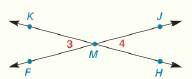Chapter 1.CR, Problem 25CR### Elementary Geometry for College St...

6th Edition
Daniel C. Alexander + 1 other
ISBN: 9781285195698

#### Solutions

Chapter
Section### Elementary Geometry for College St...

6th Edition
Daniel C. Alexander + 1 other
ISBN: 9781285195698
Textbook Problem
1 views

# Given: m ∠ 3 = 7 x − 21 m ∠ 4 = 3 x + 7 Find: m ∠ F M HTo determine

To find:

The value of mFMH.

Explanation

Given:

m3=7x21

And,

m4=3x+7

The Figure (1) is given as,

Figure (1)

Definition:

The pair of nonadjacent angles in the opposite direction that lie on two intersecting straight lines is called vertical angles.

Calculation:

Since, 3 and 4 are the vertical angles, then it can be written as,

m3=m4(1)

Substitute 7x21 for m3 and 3x+7 for m4 in equation (1).

7x21=3x+77x3x=21+74x=28x=7

The m3 can be calculated as,

m3=7x21

Substitute 7 for x in the above expression

### Still sussing out bartleby?

Check out a sample textbook solution.

See a sample solution

#### The Solution to Your Study Problems

Bartleby provides explanations to thousands of textbook problems written by our experts, many with advanced degrees!

Get Started

#### In Problems 1-8, use a calculator to evaluate each expression. 3.

Mathematical Applications for the Management, Life, and Social Sciences

#### The graph of x = cos t, y = sin2 t is:

Study Guide for Stewart's Single Variable Calculus: Early Transcendentals, 8th

#### What is the inverse of a matrix A?

Finite Mathematics for the Managerial, Life, and Social Sciences

#### Define the concept of external validity and a threat to external validity.

Research Methods for the Behavioral Sciences (MindTap Course List)